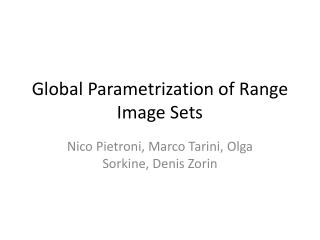DownloadDownload PresentationGlobal Parametrization of Range Image Sets

# Global Parametrization of Range Image Sets

Télécharger la présentation## Global Parametrization of Range Image Sets

- - - - - - - - - - - - - - - - - - - - - - - - - - - E N D - - - - - - - - - - - - - - - - - - - - - - - - - - -
##### Presentation Transcript

1. Global Parametrization of Range Image Sets Nico Pietroni, Marco Tarini, Olga Sorkine, Denis Zorin

2. Goal • Assign UV texture coordinate (parameterization) to 3d scanned model that consists of multiple range images Previous work • Reconstruct 3D, then parameterize Proposed method • Parameterize in each range image domain, considering constraints in overlapping area

3. Results Previous Proposed

4. Algorithms overview Parameterization Triangle area Target 3D For all triangle Expressed as least square optimization (in the form of Poisson equation) with boundary conditions How to compute w is very briefly written in section 7 Descretization and consider linearity etc. yields the following term to minimize Range UV

5. Boundary condition in overrapping area _ p Discretize, consider symmetry and linearity

6. Optimization • There are some techniques to solve this optimization (please refer to the paper)

7. Fusion of Depth Maps with Multiple Scenes Simon Fuhrmann / Michael Goesele

8. Goal • Combine registered depth maps with dramatically varying sampling rate. Solution • They introduce “scale” parameter to hierarchical signed distance octree to avoid averaging across different sampling rate.

9. Problem statement

10. Their data structure • 3D hierarchical signed distance field in octree + scale parameter • Vote triangles one by one • Also consider confidence of votes (depends on slant angle) Equation to determine the octree level Rule to update voxel values W : weight (confidence) D : distance value

11. Carefully mix different scales

12. Surface extraction • They use marching tetrahedra.

13. Reconstruction of 3D objects from arbitrary cross-section data We describe a simple algorithm to reconstruct the surface of smooth three-dimensional multi-labeled objects from sampled planar cross-sections of arbitrary orientation. The algorithm has the unique ability to handle cross-sections in which regions are classified as being inside the object, outside the object, or unknown. This is achieved by constructing a scalar function on R^3, whose zero set is the desired surface. The function is constructed independently inside every cell of the arrangement of the cross-section planes using transfinite interpolation techniques based on barycentric coordinates that guarantee that the function is smooth, and its zero set interpolates the cross-sections. Currently only abstract is available